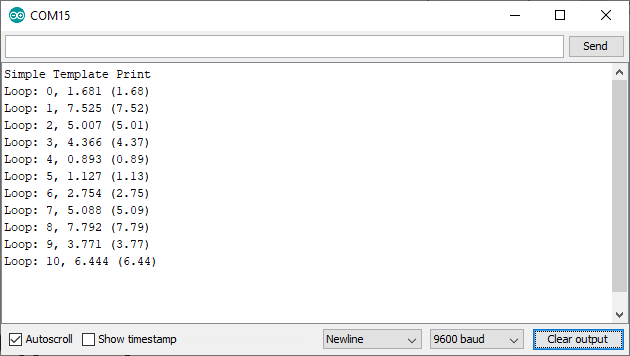# How to use the template for clear listings on the serial port

Zápisník experimentátora

To write the text to the serial port, we use the Serial object and its print function. If we need to write more information, the programming becomes an endless copy of the same command. A large number of nearly the same commands appear in the source code, with only the parameter changed. Today we'll show you how to solve this elegantly with a template.## Example

In this example, you see two functions that begin with the template keyword. This keyword tells the c++ compiler that it is not a function, but a function template. That is, the compiler generates a function based on a template based on the parameter it receives. In this example, we have two templates. The first is universal, which generates a function for the T parameter. The second is specialized only for the float type parameter. Here we list the float variable to 3 decimal places.

Another keyword that you may see for the first time is operator <<. In c++, we can also define the behavior of specialized operators that are used to perform the usual variable action. In this case, it is the << operator that is typically used to perform a bit shift. However, we can prescribe an operator's specialized behavior if its parameters are Print and T. The visual appearance of the operator suggests that we could also use it as an insert of T into Print. And that's what the function does. The first parameter of the Print type calls its `print` function with T as the parameter. The last line of the function is also important, as a result of which we return the Print object. This allows us to concatenate each operator in a row.

You can see the result in the loop function. As a result, I could use a variable of type char*, int, String, and float in a single line.

``````template<class T> inline Print& operator <<(Print &obj, T arg) {
obj.print(arg);
return obj;
}

template<> inline Print& operator <<(Print &obj, float arg) {
obj.print(arg, 3);
return obj;
}

int cnt = 0;

void setup() {
Serial.begin(9600);
Serial << "Simple Template Print\n";
}

void loop() {
float rnd = random(100000) / 10000.;
String original(rnd);
Serial << "Loop: " << cnt << ", " << rnd << " (" << original << ")\n";
cnt++;
delay(2000);
}``````

## Source code

The source code is located on the GitHub server.

12.04.2020